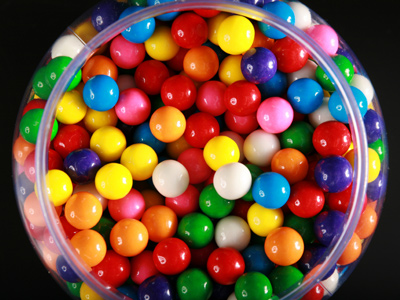In a jar of 120 sweets, one in every six sweets is orange flavoured.

# Level 5-6 Numbers - Fractions - Multiplication and Division

You will come across fractions in everyday life - half a pint of milk, quarter of an ounce of butter, an eighth of a pizza etc. Fractions are everywhere so you won't be surprised to find them in KS3 Maths.

Multiplying fractions is relatively easy. All you have to do is change any mixed numbers to top heavy fractions, then multiply the top row, and multiply the bottom row. So, to multiply 114 x 25 first change 114 to 54. Next multiply 5 x 2 and 4 x 5 to get 1020. Always look to see if you can cancel down: 1020 is the same as 12.

Dividing fractions is similar to multiplying, except you invert the second fraction, and change the divide sign to multiply. So, 114 ÷ 25 would be 54 x 52. 5 x 5 = 25 and 4 x 2 = 8 so your answer is 258 or 318.

Have a read of our Helping Children Learn Fractions page. It explains how fractions work and gives handy tips.

1.
What is 310 ÷ 625?
1
114
112
134
To divide by a fraction, invert and multiply: 3/10 ÷ 6/25 = 3/10 x 25/6. Use this method whenever you have to divide by fractions
2.
What is 3/8 of £48.00?
£6.00
£10.00
£14.00
£18.00
Rewrite the question as 3/8 x 48. 48 / 8 = 6, 6 x 3 = 18, therefore the answer is £18.00
3.
What is 234 ÷ 418?
1/3
1/2
2/3
3/4
Remember to invert the second fraction and change the divide sign to multiply
4.
What is 914 ÷ 112?
1/7
2/7
3/7
5/7
To divide by a fraction, invert and multiply
5.
14,000 fans attended the football match yesterday. 6/7 of them wore scarves. How many were without scarves?
2,000
4,000
8,000
10,000
6/7 of 14,000 = 12,000, therefore 2,000 were without scarves
6.
In a jar of 120 sweets, one in every six sweets is orange flavoured. If half the orange sweets are eaten, what fraction of the sweets is left?
56
34
1112
910
16 of 120 is 20, so 10 are eaten, leaving 110. 110120 = 1112
7.
A piece of copper wire is 723m long. What is the total length of three of these wires?
2238
23
2413
26
3 x 7 = 21 and 3 x 2/3 = 6/3 or 2 then add these two numbers together
8.
What is 214 x 215?
2310
3510
41920
512
214 becomes 94 which we multiply by 215 or 115 to get 9920 or 41920
9.
Daniel is making pancakes. Each pancake uses 18 of a litre of batter. How much batter is used if Daniel makes 20 pancakes?
212 litres
234 litres
313 litres
413 litres
I'll have lemon and sugar on mine please!
10.
What is 1/9 x 3/5?
1/12
1/14
1/15
1/18
1 x 3 = 3 and 9 x 5 = 45 so we get the answer 3/45 or 1/15